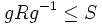# Fusion system induced by a finite group on its p-Sylow subgroup is a saturated fusion system

## Statement

Suppose$G$ is a finite group,$p$ is a prime number, and$P$ is a$p$-Sylow subgroup of$G$. Suppose$\mathcal{F}$ is defined as a category with objects the subgroups of$P$ and morphisms given as follows: For every$g \in G$ and subgroups$R,S \le P$ such that$gRg^{-1} \le S$, there is a morphism$\varphi:R \to S$ given by$\varphi(r) = grg^{-1}$. This is a category on$P$ and is in fact a fusion system.

## Proof

### Proof that it is a category

First, we prove that we get a category on$P$.

• It is closed under composition: Suppose$\alpha:R \to S$ and$\beta:S \to T$ are morphisms induced by conjugation by$g,h \in G$ respectively. Then,$gRg^{-1} \le S$ and$hSh^{-1} \le T$, so$hgRg^{-1}h^{-1} \le T$. Thus, there is a morphism in$\mathcal{F}$ from$R$ to$T$ given by conjugation by$hg$. We see that this is the composite$\beta \circ \alpha$.
• All morphisms are group homomorphisms: This follows because each morphism arises as a restriction of an inner automorphism on the whole group.
• It contains all inclusion maps: This follows because we can always set$g$ to be the identity element.
• If$\varphi:R \to S$ is a morphism, then the restriction$\varphi:R \to \varphi(R)$, and its inverse, are morphisms. This follows from the definition.

### Proof that it satisfies the conditions for a fusion system

We verify the three conditions:

• All morphisms induced by inner automorphisms from$P$ are present: This is true, because in fact all morphisms induced by inner automorphisms from$G$ are present.
• The inner automorphisms of$P$ form a$p$-Sylow subgroup of$\operatorname{Aut}_{\mathcal{F}}(P)$:$\operatorname{Aut}_{\mathcal{F}}(P)$ comprises the automorphisms induced by elements of$N_G(P)$. Since$P$ is$p$-Sylow in$G$, it is$p$-Sylow in$N_G(P)$, so since Sylow satisfies image condition, the image$\operatorname{Aut}_P(P) = \operatorname{Inn}(P)$ is$p$-Sylow in$\operatorname{Aut}_{\mathcal{F}}(P)$.
• The extension axiom: PLACEHOLDER FOR INFORMATION TO BE FILLED IN: [SHOW MORE]